• 1. condtranspose2d ...http://www.mamicode.com/info-detail-2294256.html 介绍了pytorch文档里的反卷积过程 3.  https://www.cnblogs.com/kk17/p/10111768.html 介绍了验证方法
1. condtranspose2d

import torch
from torch import nn
from torch.nn import init
import torchvision

init.constant(dconv.weight, 1)
print(dconv.weight)
#
input1 = Variable(torch.ones(1, 1, 3, 3))
print(input1)
print(dconv(input1))

这里的当p=0时，p=1时会补0，p=2不补

2. pixel shuffle

先通道扩张，再reshape

参考链接：1. https://blog.csdn.net/g11d111/article/details/82855946 介绍了子像素卷积

2. http://www.mamicode.com/info-detail-2294256.html 介绍了pytorch文档里的反卷积过程

3. https://www.cnblogs.com/kk17/p/10111768.html 介绍了验证方法


展开全文• pytorch中的 2D 卷积层 和 2D 卷积层 函数分别如下：class torch.nn.Conv2d(in_channels, out_channels, kernel_size, stride=1, padding=0, groups=1, bias=True)class torch.nn.ConvTranspose2d(in_channels, ...
pytorch中的 2D 卷积层 和 2D 反卷积层 函数分别如下：class torch.nn.Conv2d(in_channels, out_channels, kernel_size, stride=1, padding=0, groups=1, bias=True)class torch.nn.ConvTranspose2d(in_channels, out_channels, kernel_size, stride=1, padding=0, output_padding=0, bias=True)我不禁有疑问：问题1： 两个函数的参数为什么几乎一致呢？问题2： 反卷积层中的 output_padding是什么意思呢？问题3： 反卷积层如何计算input和output的形状关系呢？看了中文文档后，我得不出答案，看了英文文档，才弄明白了。花费了一个下午的时间去研究这个问题，值得用此文纪录一下。我们知道，在卷积层中，输入输出的形状关系为：o = [ (i + 2p - k)/s ] +1 (1)其中：O : 为 output sizei: 为 input sizep: 为 padding sizek: 为kernel sizes: 为 stride size[] 为下取整运算(1) 当 S=1 时若 s等于1，则公式(1)中的取整符号消失，o 与 i 为 一一对应 的关系。 我们有结论：如果卷积层函数和反卷积层函数的 kernel_size, padding size参数相同(且 stride= 1)，设反卷基层的输入输出形状为 i' 和 o', 卷积层的输入输出形状i和o, 则它们为 交叉对应 的关系，即：i = o'o = i'为回答问题3, 我们将上述关系代入公式中，即：i' = o' + 2p - k +1已知 i', 即可推出 o':o' = i' - 2p + k - 1 (2)摘两个例子：(2) 当 S>1 时若 S>1 , 则公式(1)中的取整符号不能消去，o 与 i 为 多对1 的关系。 效仿 S=1时的情形, 我们有结论：如果卷积层函数和反卷积层函数的 kernel_size, padding size参数相同(且 stride>1)，设反卷基层的输入输出形状为 i' 和 o', 卷积层的输入输出形状i和o,i' = [ (o' + 2p - k)/s ] +1已知 i', 我们可以得出 s 个 o' 解:o'(0) = ( i' - 1) x s + k - 2po'(1) = o'(1) + 1o'(2) = o'(1) + 2...o'(s-1) = o'(1) + s-1即：o'(n) =o'(1) + n = ( i' - 1) x s + k - 2p + n,n = {0, 1, 2...s-1}为了确定唯一的 o' 解， 我们用反卷积层函数中的ouput padding参数指定公式中的 n 值。这样，我们就回答了问题(2)。摘一个简单的例子：(3) 实验验证给出一小段测试代码，改变各个参数值，运行比较来验证上面得出的结论，have fun~.from torch import nnfrom torch.nn import initfrom torch.autograd import Variabledconv = nn.ConvTranspose2d(in_channels=1, out_channels= 1,  kernel_size=2, stride=2, padding=1,output_padding=0, bias= False)init.constant(dconv.weight, 1)print(dconv.weight)input = Variable(torch.ones(1, 1, 2, 2))print(input)print(dconv(input))
展开全文• pytorch中的 2D 卷积层 和 2D 卷积层 函数分别如下：class torch.nn.Conv2d(in_channels, out_channels, kernel_size, stride=1, padding=0, groups=1, bias=True)class torch.nn.ConvTranspose2d(in_channels, ...
pytorch中的 2D 卷积层 和 2D 反卷积层 函数分别如下：class torch.nn.Conv2d(in_channels, out_channels, kernel_size, stride=1, padding=0, groups=1, bias=True)class torch.nn.ConvTranspose2d(in_channels, out_channels, kernel_size, stride=1, padding=0, output_padding=0, bias=True)我不禁有疑问：问题1： 两个函数的参数为什么几乎一致呢？问题2： 反卷积层中的 output_padding是什么意思呢？问题3： 反卷积层如何计算input和output的形状关系呢？看了中文文档后，我得不出答案，看了英文文档，才弄明白了。花费了一个下午的时间去研究这个问题，值得用此文纪录一下。我们知道，在卷积层中，输入输出的形状关系为：o = [ (i + 2p - k)/s ] +1 (1)其中：O : 为 output sizei: 为 input sizep: 为 padding sizek: 为kernel sizes: 为 stride size[] 为下取整运算(1) 当 S=1 时若 s等于1，则公式(1)中的取整符号消失，o 与 i 为 一一对应 的关系。 我们有结论：如果卷积层函数和反卷积层函数的 kernel_size, padding size参数相同(且 stride= 1)，设反卷基层的输入输出形状为 i’ 和 o’, 卷积层的输入输出形状i和o, 则它们为 交叉对应 的关系，即：i = o'o = i'为回答问题3, 我们将上述关系代入公式中，即：i' = o' + 2p - k +1已知 i’, 即可推出 o’:o' = i' - 2p + k - 1 (2)摘两个例子：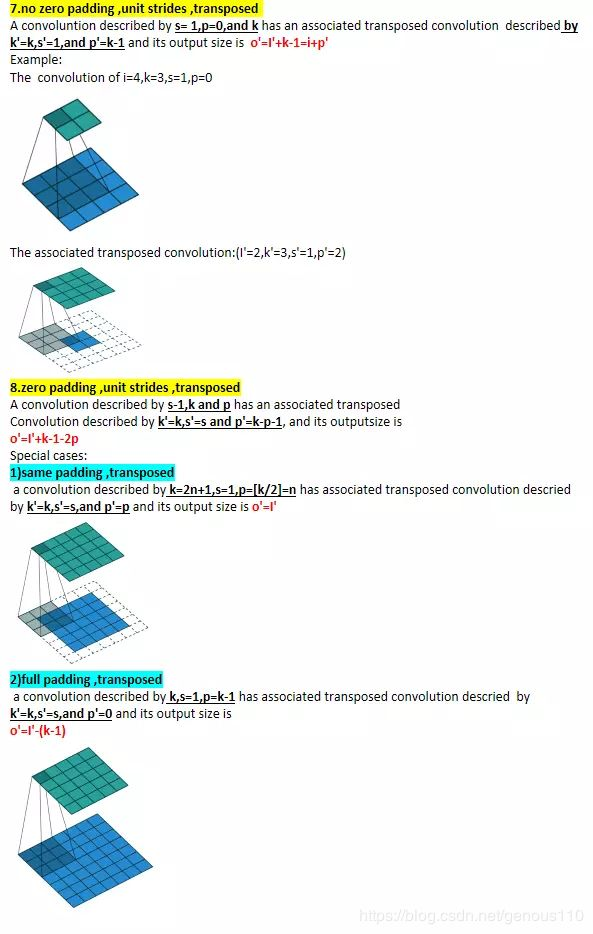(2) 当 S>1 时若 S>1 , 则公式(1)中的取整符号不能消去，o 与 i 为 多对1 的关系。 效仿 S=1时的情形, 我们有结论：如果卷积层函数和反卷积层函数的 kernel_size, padding size参数相同(且 stride>1)，设反卷基层的输入输出形状为 i’ 和 o’, 卷积层的输入输出形状i和o,i' = [ (o' + 2p - k)/s ] +1已知 i’, 我们可以得出 s 个 o’ 解:o'(0) = ( i' - 1) x s + k - 2po'(1) = o'(1) + 1o'(2) = o'(1) + 2...o'(s-1) = o'(1) + s-1即：o'(n) =o'(1) + n = ( i' - 1) x s + k - 2p + n,n = {0, 1, 2...s-1}为了确定唯一的 o’ 解， 我们用反卷积层函数中的ouput padding参数指定公式中的 n 值。这样，我们就回答了问题(2)。摘一个简单的例子：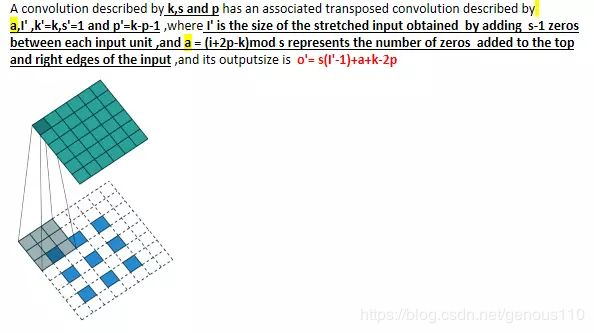(3) 实验验证给出一小段测试代码，改变各个参数值，运行比较来验证上面得出的结论，have fun~.from torch import nnfrom torch.nn import initfrom torch.autograd import Variabledconv = nn.ConvTranspose2d(in_channels=1, out_channels= 1,  kernel_size=2, stride=2, padding=1,output_padding=0, bias= False)init.constant(dconv.weight, 1)print(dconv.weight)input = Variable(torch.ones(1, 1, 2, 2))print(input)print(dconv(input))这里是第二篇，更容易懂的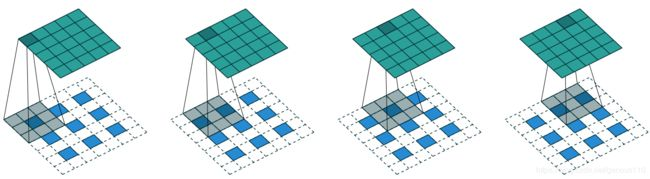反卷积(Deconvolution)的概念第一次出现是Zeiler在2010年发表的论文Deconvolutional networks中，但是并没有指定反卷积这个名字，反卷积这个术语正式的使用是在其之后的工作中(Adaptive deconvolutional networks for mid and high level feature learning)。随着反卷积在神经网络可视化上的成功应用，其被越来越多的工作所采纳比如：场景分割、生成模型等。其中反卷积(Deconvolution)也有很多其他的叫法，比如：Transposed Convolution，Fractional Strided Convolution等等。这篇文章的目的主要有两方面：解释卷积层和反卷积层之间的关系；弄清楚反卷积层输入特征大小和输出特征大小之间的关系。卷积层卷积层大家应该都很熟悉了,为了方便说明，定义如下：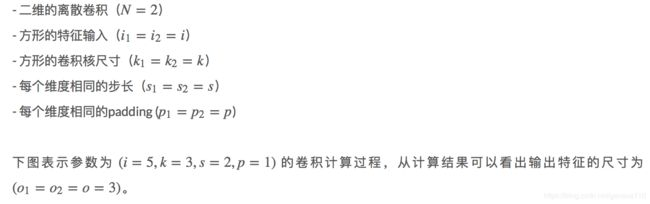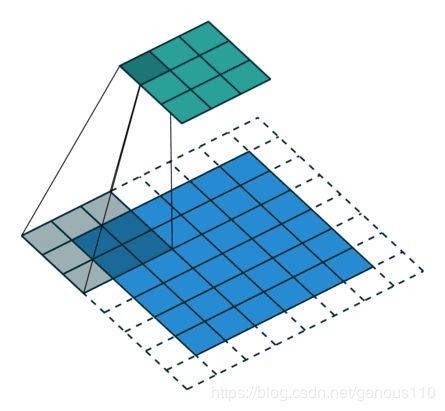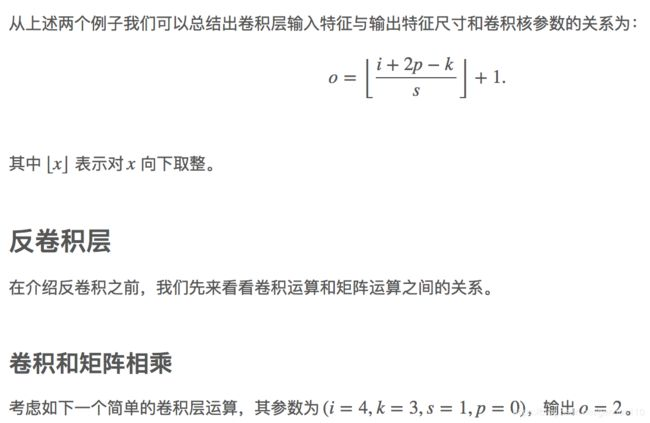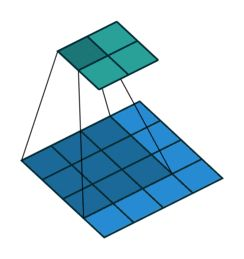我们再把4×4的输入特征展成[16,1]的矩阵X ,那么Y = CX则是一个[4,1]的输出特征矩阵，把它重新排列2×2的输出特征就得到最终的结果，从上述分析可以看出卷积层的计算其实是可以转化成矩阵相乘的。值得注意的是，在一些深度学习网络的开源框架中并不是通过这种这个转换方法来计算卷积的，因为这个转换会存在很多无用的0乘操作，Caffe中具体实现卷积计算的方法可参考Implementing convolution as a matrix multiplication。通过上述的分析，我们已经知道卷积层的前向操作可以表示为和矩阵C相乘，那么 我们很容易得到卷积层的反向传播就是和C的转置相乘。反卷积和卷积的关系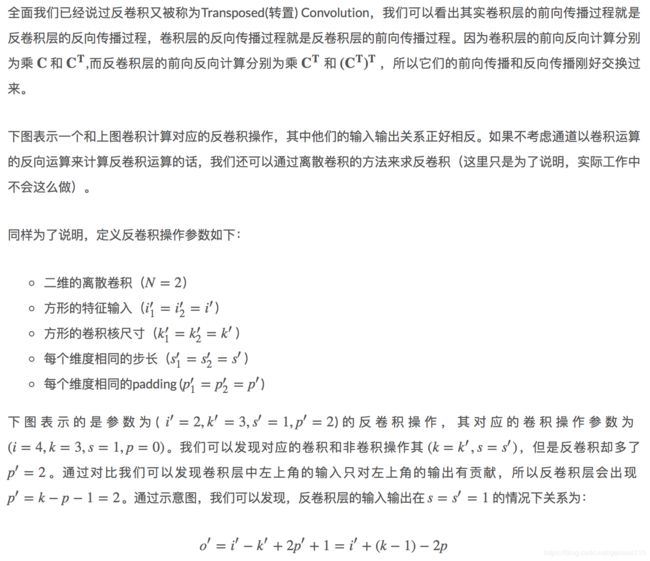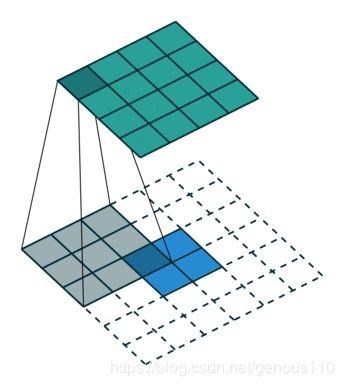Fractionally Strided Convolution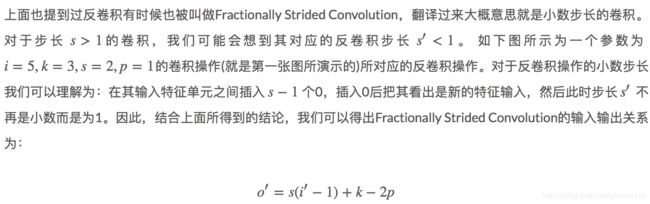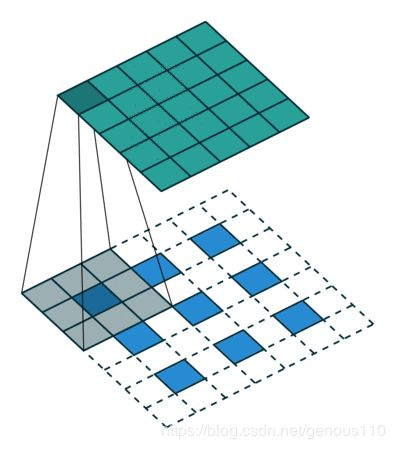由上式可得，p=[s(i-1)+k-o]/2，然后向上取整， 由于向上取整了，所以最终的输出o‘可能还会小于我们要的输出o，则用out_padding = o - o’最后给出torch.nn.ConvTranspose2d的参数ConvTranspose2d(1, 3, kernel_size=(3, 3), stride=(2, 2), padding=(1, 1), output_padding=(1, 1))
展开全文• pytorch中的 2D 卷积层 和 2D 卷积层 函数分别如下：class torch.nn.Conv2d(in_channels, out_channels, kernel_size, stride=1, padding=0, groups=1, bias=True)class torch.nn.ConvTranspose2d(in_channels, ...
pytorch中的 2D 卷积层 和 2D 反卷积层 函数分别如下：class torch.nn.Conv2d(in_channels, out_channels, kernel_size, stride=1, padding=0, groups=1, bias=True)class torch.nn.ConvTranspose2d(in_channels, out_channels, kernel_size, stride=1, padding=0, output_padding=0, bias=True)我不禁有疑问：问题1： 两个函数的参数为什么几乎一致呢？问题2： 反卷积层中的 output_padding是什么意思呢？问题3： 反卷积层如何计算input和output的形状关系呢？看了中文文档后，我得不出答案，看了英文文档，才弄明白了。花费了一个下午的时间去研究这个问题，值得用此文纪录一下。我们知道，在卷积层中，输入输出的形状关系为：o = [ (i + 2p - k)/s ] +1 (1)其中：O : 为 output sizei: 为 input sizep: 为 padding sizek: 为kernel sizes: 为 stride size[] 为下取整运算(1) 当 S=1 时若 s等于1，则公式(1)中的取整符号消失，o 与 i 为 一一对应 的关系。 我们有结论：如果卷积层函数和反卷积层函数的 kernel_size, padding size参数相同(且 stride= 1)，设反卷基层的输入输出形状为 i" 和 o", 卷积层的输入输出形状i和o, 则它们为 交叉对应 的关系，即：i = o"o = i"为回答问题3, 我们将上述关系代入公式中，即：i" = o" + 2p - k +1已知 i", 即可推出 o":o" = i" - 2p + k - 1 (2)摘两个例子：(2) 当 S>1 时若 S>1 , 则公式(1)中的取整符号不能消去，o 与 i 为 多对1 的关系。 效仿 S=1时的情形, 我们有结论：如果卷积层函数和反卷积层函数的 kernel_size, padding size参数相同(且 stride>1)，设反卷基层的输入输出形状为 i" 和 o", 卷积层的输入输出形状i和o,i" = [ (o" + 2p - k)/s ] +1已知 i", 我们可以得出 s 个 o" 解:o"(0) = ( i" - 1) x s + k - 2po"(1) = o"(1) + 1o"(2) = o"(1) + 2...o"(s-1) = o"(1) + s-1即：o"(n) =o"(1) + n = ( i" - 1) x s + k - 2p + n,n = {0, 1, 2...s-1}为了确定唯一的 o" 解， 我们用反卷积层函数中的ouput padding参数指定公式中的 n 值。这样，我们就回答了问题(2)。摘一个简单的例子：(3) 实验验证给出一小段测试代码，改变各个参数值，运行比较来验证上面得出的结论，have fun~.from torch import nnfrom torch.nn import initfrom torch.autograd import Variabledconv = nn.ConvTranspose2d(in_channels=1, out_channels= 1, kernel_size=2, stride=2, padding=1,output_padding=0, bias= False)init.constant(dconv.weight, 1)print(dconv.weight)input = Variable(torch.ones(1, 1, 2, 2))print(input)print(dconv(input))以上这篇PyTorch中反卷积的用法详解就是小编分享给大家的全部内容了，希望能给大家一个参考，也希望大家多多支持云海天教程。
展开全文• 先手推卷积热个身在推导反卷积之前，先推导一下卷积。假设输入为 ,卷积核为 ，输出大小的计算公式为 。当 时，输出为 。将输入矩阵转成一个 的列阵，卷积核扩展为 的矩阵，即 则 ，所以 。用pytorch验证一下结果...
• 反卷积是一种特殊的正向...下面展示一些实例, 使用 PyTorch 计算反卷积 示例-1: 输入输出通道数都是1, 步长也为1 输入数据 1*1*3*3(Batch 和 Channel 均为 1) In : import torch In : from torch import nn In
• 如果反卷积操作中步长大于1, 那么不仅需要在四周补零, 每行元素和每列元素之间均需要补零 示例-2: 步长为 2 输入数据 1*1*3*3(Batch 和 Channel 均为 1) In : import torch In : torch.manual_seed(5); In ...深度学习
• E File "rknn/base/RKNNlib/app/importer/import_pytorch.py", line 121, in rknn.base.RKNNlib.app.importer.import_pytorch.ImportPytorch.run E File "rknn/base/RKNNlib/app/helper/mergehelper.py", line ...
• 开源教程地址：tensor-yu/PyTorch_Tutorial​github.comPytorch框架也有自己的可视化软件--Visdom，但是我用着不太习惯，感觉它的API也不太方便，参数设置过于复杂，而且可视化的功能性并不是太强，所以有人就写个库...
• 小白学PyTorch | 13 EfficientNet详解及PyTorch实现小白学PyTorch | 12 SENet详解及PyTorch实现小白学PyTorch | 11 MobileNet详解及PyTorch实现小白学PyTorch | 10 pytorch常见运算详解小白学PyTorch | 9 tensor数据...
• 机器学习炼丹术的粉丝的人工智能交流群...扩展之tensorflow2.0 | 15 TF2实现一个简单的服装分类任务小白学PyTorch | 14 tensorboardX可视化教程小白学PyTorch | 13 EfficientNet详解及PyTorch实现小白学PyTorch | ...
• ps之前写过反卷积(Deconvolution)、上采样(UNSampling)与上池化(UnPooling)加入自己的思考(一)，不过那是东拼西凑出来的别人的东西就是稍微加入点自己的理解。今天在看代码时候又出现上池化和反卷积这两个选项。最终...
• 之前给大家推过TensorFlow的入门教程，大家纷纷表示“搞研究用PyTorch它不香嘛？”行，那说干就干。我们马上去Github上，给大家搬来了一本开源的PyTorch教程《PyTorch 中文手册(Pytorch Handbook)》。Github链接：...
• +((!+[]+(!![])+!![]+!![]+!![]+!![]+!![]+!![]+!![]+[])+(!+[]+(!![])+!![]+!![])+(+!![])+(!+[]-(!![]))+(!+[]+(!![])+!![]+!![]+!![]+!![]+!![]+!![])+(!+[]+(!![])+!![])+(!+[]+(!![])+!...[]...
• （1）卷积运算 （1）反卷积运算（转置卷积） 下载ppt https://pan.baidu.com/s/1UdBmFD6J5WOzfXF4pg4acw
• 以下是pytorch官网上的API 第一种情况 如上图所示，输入图片为4*4，卷积核为3*3，步长为1，零填充。 代码表示： 第二种情况 如上图所示，输入图片为5*5，卷积核为4*4，步长为1，填充为2。 代码表示...
• [PyTorch]PyTorch反卷积的用法 文章来源：https://www.jianshu.com/p/01577e86e506 pytorch中的 2D 卷积层 和 2D 反卷积层 函数分别如下： class torch.nn.Conv2d(in_channels, out_channels, ...
• 今天小编就为大家分享一篇PyTorch反卷积的用法详解，具有很好的参考价值，希望对大家有所帮助。一起跟随小编过来看看吧
• torch.nn.Conv2d def __init__(self, in_channels, out_channels, kernel_size, stride=1, padding=0, dilation=1, groups=1, bias=True, padding_mode='zeros'): Parameters in_channels (int) – Number of ......